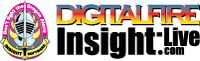•The secret to cool bodies and glazes is a lot of testing.
•The secret to know what to test is material and chemistry knowledge.
•The secret to learning from testing is documentation.
•The place to test, do the chemistry and document is an account at https://insight-live.com
•The place to get the knowledge is https://digitalfire.com

Sign-up at https://insight-live.com today.

Rice Straw Ash

 Oxide Analysis Formula CaO 2.74% 0.352 MgO 1.49% 0.266 K2O 3.29% 0.252 Na2O 0.56% 0.065 P2O5 1.29% 0.065 Al2O3 10.78% 0.761 SiO2 77.26% 9.258 Fe2O3 0.53% 0.024 MnO 0.70% 0.071 SO3 1.35 Oxide Weight 710.34 Formula Weight 720.06

XML for Import into INSIGHT

<?xml version="1.0" encoding="UTF-8"?> <material name="Rice Straw Ash" descrip="" searchkey="" loi="0.00" casnumber=""> <oxides> <oxide symbol="CaO" name="Calcium Oxide, Calcia" status="" percent="2.740" tolerance=""/> <oxide symbol="MgO" name="Magnesium Oxide, Magnesia" status="" percent="1.490" tolerance=""/> <oxide symbol="K2O" name="Potassium Oxide" status="" percent="3.290" tolerance=""/> <oxide symbol="Na2O" name="Sodium Oxide, Soda" status="" percent="0.560" tolerance=""/> <oxide symbol="P2O5" name="Phosphorus Pentoxide" status="" percent="1.290" tolerance=""/> <oxide symbol="Al2O3" name="Aluminum Oxide, Alumina" status="" percent="10.780" tolerance=""/> <oxide symbol="SiO2" name="Silicon Dioxide, Silica" status="" percent="77.260" tolerance=""/> <oxide symbol="Fe2O3" name="Iron Oxide, Ferric Oxide" status="" percent="0.530" tolerance=""/> <oxide symbol="MnO" name="Manganous Oxide" status="" percent="0.700" tolerance=""/> </oxides> <volatiles> <volatile symbol="SO3" name="Sulfur Trioxide" percent="1.350" tolerance=""/> </volatiles> </material>